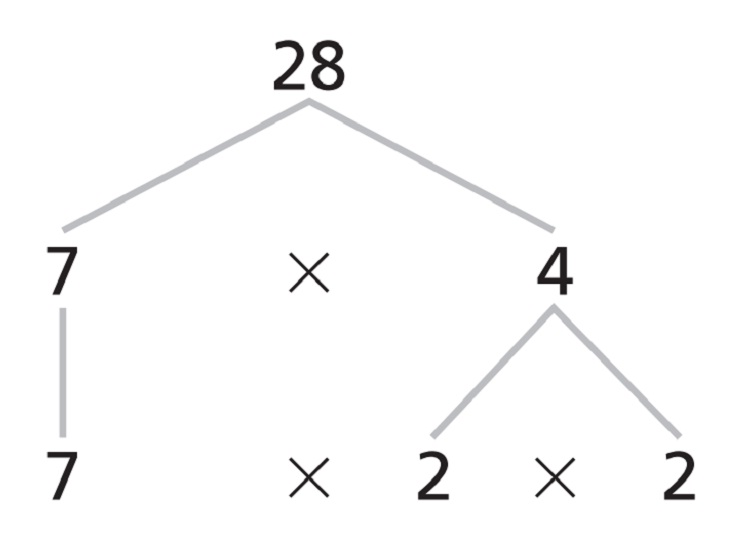﻿ Prime Factorization Calculator | Prime Factor Calculator Online

# Prime Factors Calculator

Use our prime factors calculator, prime factorization calculator, a free online conversion tool to calculate product of prime factor of a number.

## Definition and Facts About Prime Factorization

A prime number is a whole number greater than one not divisible without a remainder by any whole number other than itself and one. The prime factors of a value are all of the prime numbers that will exactly divide the given value. The prime factorization of a composite number is a factorization that contains only prime numbers. The Prime Factorization of a number is the product of prime factors equal to that number. Many proofs in number theory make use of the following important theorem.

#### Every composite number can be written as a unique product of prime numbers

To find the prime factorization of a composite number, rewrite the number as a product of two smaller natural numbers. If these smaller numbers are both prime numbers, then you are finished. If either of the smaller numbers is not a prime number, then rewrite it as a product of smaller natural numbers. Continue this procedure until all factors are primes.

#### Examples : How to find Prime Factor

You can use factor trees to find the prime factorization of a number. For example, to make a factor tree for 28, begin by choosing two numbers whose product is 28. Continue dividing each number into two factors untileach of the branches ends in a prime number. The prime factorization of 28 is 2 x 2 x 7, or 22 x 7.### Spreading Knowledge Across the World

USA - United States of America  Canada  United Kingdom  Australia  New Zealand  South America  Brazil  Portugal  Netherland  South Africa  Ethiopia  Zambia  Singapore  Malaysia  India  China  UAE - Saudi Arabia  Qatar  Oman  Kuwait  Bahrain  Dubai  Israil  England  Scotland  Norway  Ireland  Denmark  France  Spain  Poland  and  many more....